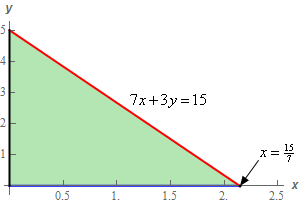Paul's Online Notes
Home / Calculus III / Surface Integrals / Parametric Surfaces
Show Mobile Notice Show All Notes Hide All Notes
Mobile Notice
You appear to be on a device with a "narrow" screen width (i.e. you are probably on a mobile phone). Due to the nature of the mathematics on this site it is best views in landscape mode. If your device is not in landscape mode many of the equations will run off the side of your device (should be able to scroll to see them) and some of the menu items will be cut off due to the narrow screen width.

### Section 17.2 : Parametric Surfaces

2. Write down a set of parametric equations for the plane $$7x + 3y + 4z = 15$$ that lies in the 1st octant.

Show All Steps Hide All Steps

Start Solution

This problem is really just an extension of the previous problem so we’ll redo the set of parametric equations for the plane a little quicker this time.

First, we need to solve the equation for any of the three variables. We’ll solve for $$z$$ in this case to get,

$z = \frac{{15}}{4} - \frac{7}{4}x - \frac{3}{4}y$

The parametric equation for this plane is then,

$\vec r\left( {x,y} \right) = \left\langle {x,y,z} \right\rangle = \left\langle {x,y,\frac{{15}}{4} - \frac{7}{4}x - \frac{3}{4}y} \right\rangle$

Remember that all we need to do to get the parametric equations is plug in the equation for $$z$$ into the $$z$$ component of the vector $$\left\langle {x,y,z} \right\rangle$$.

Show Step 2

Now, the set of parametric equations from above is for the full plane and that isn’t what we want in this problem. In this problem we only want the portion of the plane that is in the 1st octant.

So, we’ll need to restrict $$x$$ and $$y$$ so that the parametric equation from Step 1 will only give the portion of the plane that is in the 1st octant.

If you recall how to get the region $$D$$ for a triple integral then you know how to do this because it is basically the same idea. In this case we need the region $$D$$ in the $$xy$$-plane that will give the plane in the 1st octant.

Here is a sketch of this region.The hypotenuse is just where the plane intersects the $$xy$$-plane and so we can quickly find the equation of the line by setting $$z = 0$$ in the equation of the plane.

We can either solve this for $$x$$ or $$y$$ to get the ranges for $$x$$ and $$y$$. It doesn’t really matter which we solve for here so let’s just solve for $$y$$ to get the following ranges for $$x$$ and $$y$$ to describe this triangle.

$\begin{array}{c} \displaystyle 0 \le x \le \frac{{15}}{7}\\ \displaystyle 0 \le y \le - \frac{7}{3}x + 5\end{array}$

Putting this all together we get the following set of parametric equations for the plane that is in the 1st octant.

$\require{bbox} \bbox[2pt,border:1px solid black]{{\vec r\left( {x,y} \right) = \left\langle {x,y,\frac{{15}}{4} - \frac{7}{4}x - \frac{3}{4}y} \right\rangle \,\,\,\,\,\,\,\,\,0 \le x \le \frac{{15}}{7}\,\,\,,\,\,0 \le y \le - \frac{7}{3}x + 5}}$